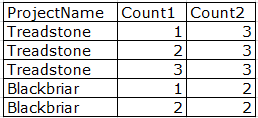# How to count the number of features with the same value for an attribute

5682
11
01-16-2018 09:15 AMNew Contributor

I need a way in python to have the Count1 (in the image).I have already did it using excel, but due to the client requirement it must be done either in modelbuilder or in a python scrit, i currently have the script to have the Count2, so i only need the Count1

Note: the data displayed in the image is not the actual data but a example)

Tags (3)
1 Solution

Accepted SolutionsbyEsri Esteemed Contributor

In case you have a featureclass (or table) with only the Project name info and the output fields (count1 and count2) already present in the featureclass you could do something like this:

``````def main():
import arcpy
tbl = r'C:\Identity\Supremacy\Ultimatun\Legacy\Bourne' # path to table or featureclass
fld_proj = 'ProjectName'
fld_cnt1 = 'Count1'
fld_cnt2 = 'Count2'

# update cycle 1 (asuming data is sorted)
flds = (fld_proj, fld_cnt1)
prev_proj = None
cnt = 0
dct = {}
with arcpy.da.UpdateCursor(tbl, flds) as curs:
for row in curs:
proj = row
if proj != prev_proj:
seq = 1
else:
seg += 1
dct[proj] = seq
row = seq
curs.updateRow(row)

# update cycle 2
flds = (fld_proj, fld_cnt2)
with arcpy.da.UpdateCursor(tbl, flds) as curs:
for row in curs:
proj = row
if proj in dct:
row = dct[proj]
else:
# this should not happen
row = -1
curs.updateRow(row)

if __name__ == '__main__':
main()‍‍‍‍‍‍‍‍‍‍‍‍‍‍‍‍‍‍‍‍‍‍‍‍‍‍‍‍‍‍‍‍‍‍‍‍‍``````
11 RepliesbyMVP Legendary Contributor

Are they always sequential? or are they randomly mixed?

This does sequential

``````old = ""
cnt = 0
def seq_count(val):
global old
global cnt
if old == val:
cnt += 1
ret = cnt
else:
cnt = 0
ret = cnt
old = val
return ret‍‍‍‍‍‍‍‍‍‍‍‍‍``````New Contributor

this code is for the field calculator?byMVP Legendary Contributor

you can use it in the field calculator... hence you can also use it as the codeblock for the Calculate field as indicatedbyMVP Esteemed Contributor

I can read your question a couple differnet ways.  What is the expected outcome for your example?  Do you want to know how many features have a Count1 value of 1, Count1 value of 2, etc... or are you interested in how many features have a total of 2 count values in Count1, 3 count values in Count3?New Contributor

i'm interested in getting the Count1 column, which gives sequential numbers for those features with the same ProjectName.

The values are already sorted, so all the features with the same ProjectName are already as displayed in the image.

At the end i will have many features with Count1=1, Count1=2, etc. but it doesnt matterbyMVP Legendary Contributor

try my posted code then.  It can be implemented as a code block for the Calculate Field tool for use in arcpy or modelbuilderbyMVP Esteemed Contributor

Ah, you are asking a different question entirely than what I first thought.  Besides Dan's code here, different flavors of the same question have been asked several times over the past couple years on GeoNet:  Calculating counting field , https://community.esri.com/thread/200080-is-it-possible-to-use-a-group-by-function-in-the-arcpy-upda...New Contributor

Tried a slight variation of the code in the link you sugested and worked perfectly thanksbyMVP Frequent Contributor

Like what Joshua Bixby suggested, the question can be read many ways, which complicates finding a solution.  Can you restate what you need in a different way so we can get a better understanding of what the goal is?

As for a solution, so far it is sounding like what possibly would work is a Summarize (Modelbuilder) or its Python equivalent.

Summarizing data in a table—Help | ArcGIS for Desktop   However, that is just a guess based on what most of the possible goals could be.

Chris Donohue, GISP